# Motion with Acceleration

This topic is part of the HSC Physics course under the section Motion in a Straight Line.

### HSC Physics Syllabus

• conduct practical investigations, selecting from a range of technologies, to record and analyse the motion of objects in a variety of situations in one dimension in order to measure or calculate:

– time
– distance
– displacement
– speed
– velocity
– acceleration
• use mathematical modelling and graphs, selected from a range of technologies, to analyse and derive relationships between time, distance, displacement, speed, velocity and acceleration in rectilinear motion, including:
– s=ut+1/2at^2
– v=u+at
– v^2=u^2+2as (ACSPH061)

### Acceleration

Motion is the change of position of an object with respect to a reference point over time. Acceleration is the rate at which an object's velocity changes with respect to time.

Acceleration is a vector quantity, meaning it has both magnitude and direction. It is usually represented by the symbol "a" and its unit of measurement is meters per second squared (m s^{-2}). This means that for every second an object accelerates, its speed increases by a certain number of meters per second.

There are two types of acceleration: positive and negative.

### Positive Acceleration

Positive acceleration, also known as acceleration in the positive direction, causes an object's velocity to increase. The effect of positive acceleration of an object's speed depends on its direction of motion.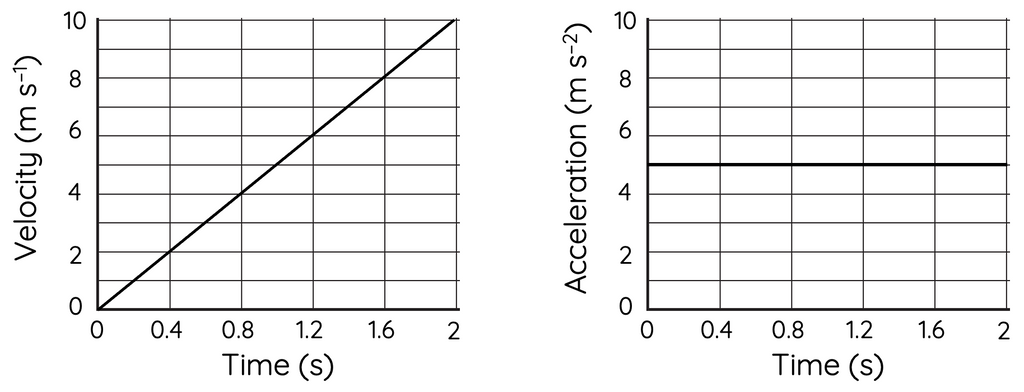If an object with positive velocity experiences positive acceleration, its velocity and thus, speed will increase over time.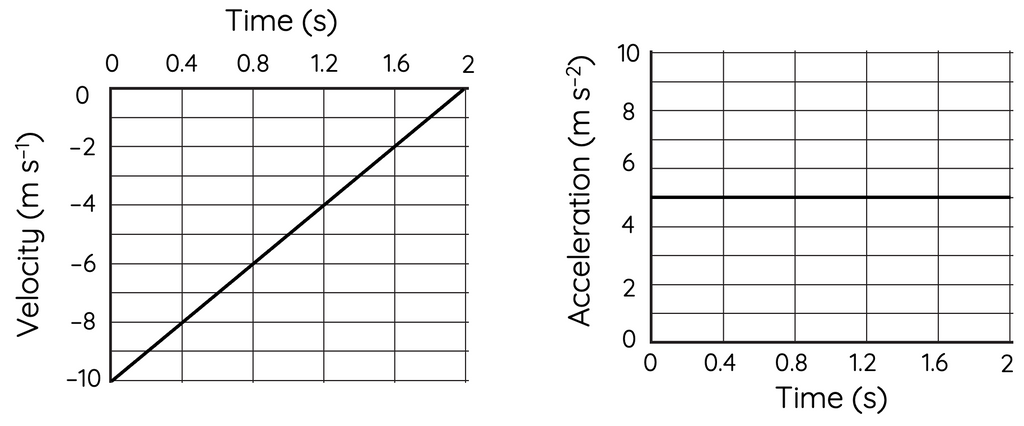If an object with negative velocity experiences positive acceleration, its velocity will increase (becomes less negative), and therefore its speed will decrease over time.

### Negative Acceleration

Negative acceleration, also known as deceleration or acceleration in the negative direction, causes an object's velocity to decrease. The effect of negative acceleration on an object's speed depends on its direction of motion.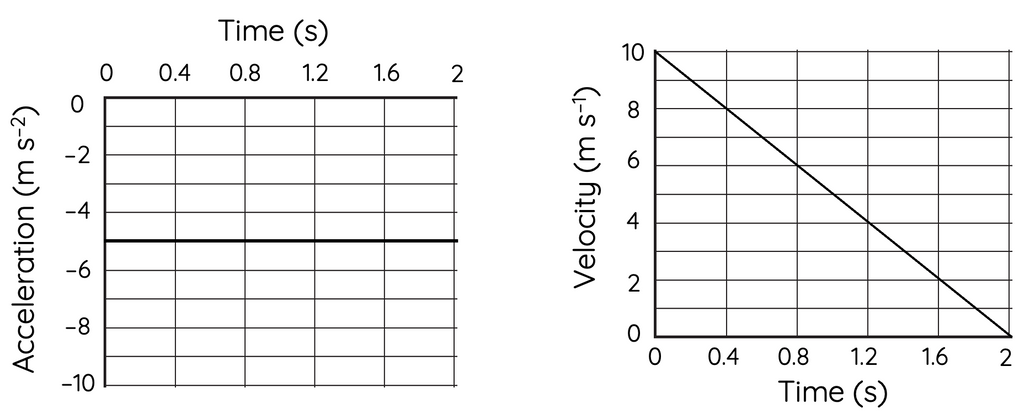If an object with positive velocity experiences negative acceleration, its velocity and speed will decrease over time.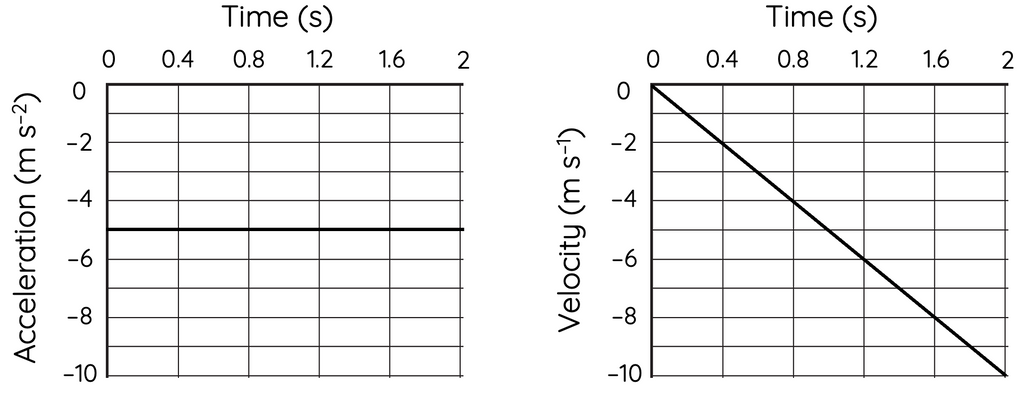If an object with negative velocity experiences negative acceleration, its velocity will decrease (becomes more negative). As a result, its speed will increase over time.

### Graphs of Motion with Constant Acceleration

The graphs of motion with constant acceleration are an important tool for understanding the motion of an object.

### Acceleration vs. time graph

The acceleration vs. time graph represents an object's acceleration as a function of time. If the object is moving with constant acceleration, the acceleration vs. time graph will be a horizontal line (see above). The slope of the line is zero, which indicates that the acceleration is constant.

### Velocity vs. time graph

The velocity vs. time graph represents an object's velocity as a function of time. If the object is moving with constant acceleration, the velocity vs. time graph will be a straight line. The slope or gradient of the line represents the object's acceleration, while the area under the curve represents the object's displacement.• If the slope is positive, the object is moving in the positive direction (i.e. its velocity is increasing)
• If the slope is negative, the object is moving in the negative direction (i.e. its velocity is decreasing).

### Displacement vs. time graph

The displacement vs. time graph represents an object's position as a function of time. If the object is moving with constant acceleration, the displacement vs. time graph will be a parabolic curve. The shape of the curve depends on the initial position, initial velocity, and acceleration of the object.

• The slope of the curve represents the object's velocity at any given time (instantaneous velocity).
• The area under the curve represents the object's displacement.The displacement vs time graph above demonstrates the motion of an object with positive velocity and positive acceleration. The displacement increases (becomes more positive) over time which suggests the object is moving in the positive direction (positive velocity). The slope of the curve increases with time, which suggests the velocity increases with time. This in turn suggests the object as a positive acceleration.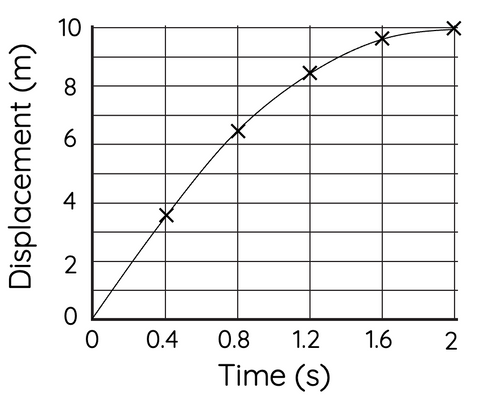The displacement vs time graph above demonstrates the motion of an object with positive velocity and negative acceleration. The slope of the curve decreases with time which suggests the velocity and speed are decreasing. This in turn suggests the acceleration of the object is negative (opposite to velocity).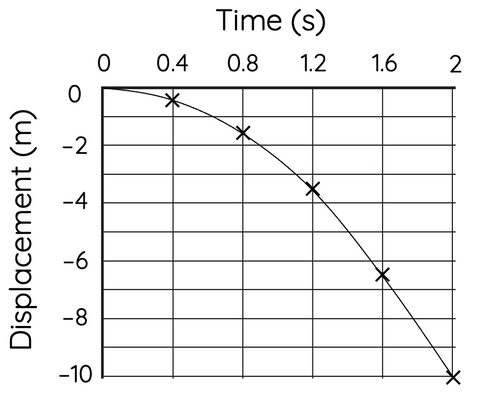This displacement vs time graph demonstrates the motion of an object with negative velocity and negative acceleration. The displacement decreases (becomes more negative) which suggests the object is moving in the negative direction (negative velocity). Since the curve become steeper with time, the velocity becomes increasing more negative (speed increases). This in turn suggests the acceleration is negative (same direction as velocity).